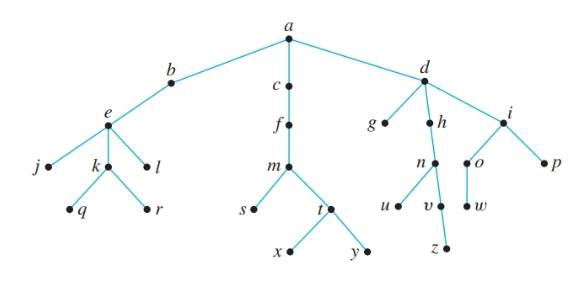Chapter 10.5, Problem 1ES### Discrete Mathematics With Applicat...

5th Edition
EPP + 1 other
ISBN: 9781337694193

#### Solutions

Chapter
Section### Discrete Mathematics With Applicat...

5th Edition
EPP + 1 other
ISBN: 9781337694193
Textbook Problem
1 views

# Consider the tree shown below with root a. a. What is the level of n? b. What is the level of a? c. What is the height of this rooted tree? d. What are the children of n? e. What is the parent of g? f. What are the siblings of j? g. What are the descendants of f? h. How many leaves (terminal vertices) are on the tree?To determine

(a)

To find:

Level of n.

Explanation

Given information:

Calculation:

The level of a vertex is the length of the path from the root to the vertex...

To determine

(b)

To find:

Level of a.

To determine

(c)

To find:

height of the rooted tree.

To determine

(d)

To find:

children of n.

To determine

(e)

To find:

Parent of g.

To determine

(f)

To find:

Siblings of j.

To determine

(g)

To find:

Descendants of f.

To determine

(h)

To find:

Number of leaves (terminal vertices) on the tree.

### Still sussing out bartleby?

Check out a sample textbook solution.

See a sample solution

#### The Solution to Your Study Problems

Bartleby provides explanations to thousands of textbook problems written by our experts, many with advanced degrees!

Get Started